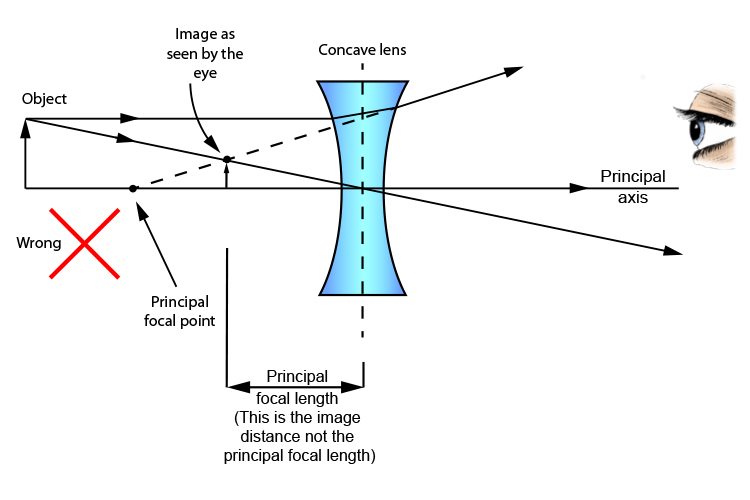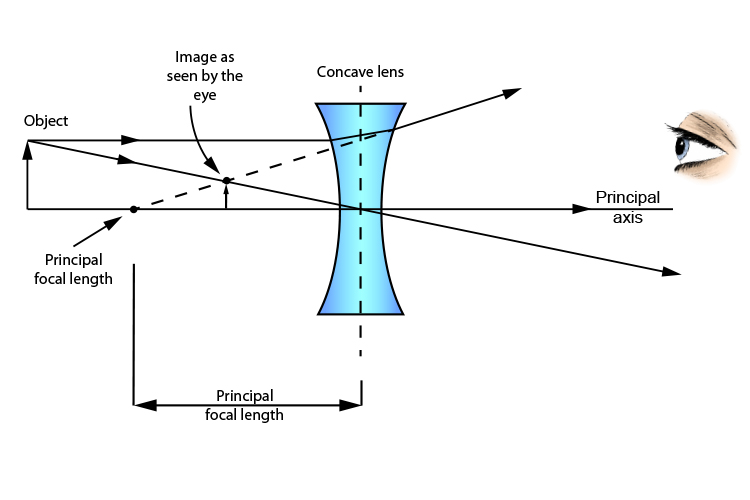# Concave lens: Principal focal length – be careful

To compare one concave lens with another you need to know the focal length of each lens.

Some books define the focal length of a concave lens as the distance from the centre of the lens to where the image will appear to a viewer the other side of the lens. But this is not correct, this is the "image distance" not the principal focal length.The focal length of a concave lens is only the distance between the centre of the concave lens and the principal focal point and should always be called the principal focal length. See below: